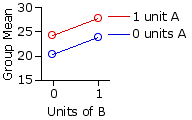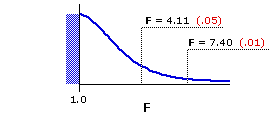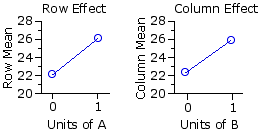Chapter 16.
Two-Way Analysis of Variance for Independent Samples
Part 2

• Example 1.

 rawdata B 0 units 1 unit A 0units 20.4 17.4   20.0 18.4   24.5 21.0   19.7 22.3   17.3 23.3 20.5 26.3   26.6 19.8   25.4 28.2   22.6 23.7   22.5 22.6 1 unit 22.4 19.1   22.4 25.4   26.2 25.1   28.8 21.8   26.3 25.2 34.1 21.9   32.6 28.5   29.0 25.8   29.0 27.1   25.7 24.4
In order to test the separate and mutual effects of two drugs, A and B, on physiological arousal, researchers randomly and independently sorted 40 laboratory rats into four groups of 10 subjects each. Each group received a certain dosage of drug A (zero units or 1 unit) and a certain dosage of drug B (zero units or 1 unit). The dependent variable was a standard measure of physiological arousal. As in the earlier two-drug illustration, one of the groups served as a control, receiving only an inert placebo containing zero units of A and zero units of B. The adjacent table shows the consequent measures of physiological arousal for each subject in each of the four groups.

Performing the requisite number-crunching on this array yields the summary values shown in the next table. The values pertaining to the four groups of measures in the original table of raw data are subscripted as g1, g2, g3, and g4; those pertaining to the rows are subscripted as r1 and r2; and those pertaining to the columns are subscripted as c1 and c2. The values deriving from the entire array of data (all groups combined) are as usual subscripted "T." It is worth a moment of your time to look back and forth between the table above and the one below to make sure you have a clear sense of where the summary values are coming from. (Click here if you would like a printable summary of these and other relevant tables of information as you work your way through this example.)

 summarydata B 0 units 1 unit rows A 0units Ng1=10 ∑Xg1=204.3 ∑X2g1=4226.3 Ng2=10 ∑Xg2=238.2 ∑X2g2=5741.4 Nr1=20 ∑Xr1=442.5 1 unit Ng3=10 ∑Xg3=242.7 ∑X2g3=5961.34 Ng4=10 ∑Xg4=278.1 ∑X2g4=7855.3 Nr2=20 ∑Xr2=520.8 columns Nc1=20 ∑Xc1=447.0 Nc2=20 ∑Xc2=516.3 NT=40 ∑XT=963.3 ∑X2T=23784.4

Up to this point it is all a tangle of numbers with no immediately discernible structure. It is only when you calculate the various means—for groups, rows, columns, and total array of data—that the pattern becomes visible. These means are given in the next table, along with a plot of the group means analogous to the ones shown in the earlier illustrations.

 means B 0 units 1 unit rowsA 0units Mg1=20.43 Mg2=23.82 Mr1=22.13 1 unit Mg3=24.27 Mg4=27.81 Mr2=26.04 columns Mc1=22.35 Mc2=25.82 MT=24.08

The resemblance between the above graph and the plot of our earlier Scenario 2 is no accident. When doing actual research, you must of course take your data as they come. The nice thing about generating illustrative data for a textbook is that you can shape them in any way you want. For this example I have deliberately arranged things so that there will be main effects for the row and column variables, but no interaction effect. The rationale is that understanding the presence of an interaction effect is best arrived at by first understanding its absence.

The next table shows the sums of squared deviates within each of the four groups, as well as for all four groups combined. These values are calculated exactly as they are in the corresponding one-way ANOVA, according to the general computational formula
 SS = ∑X2i — (∑Xi)2N

 preliminarySS values B 0 units 1 unit A 0units SSg1=52.44 SSg2=67.48 1 unit SSg3=71.02 SSg4=121.37 SST=585.70

Also as in the one-way ANOVA, SSwg is given by the sum of the SS measures within the several groups. In the present case there are four groups; hence

 SSwg = SSg1 + SSg2 + SSg3 + SSg4 = 52.44 + 67.48 + 71.02 + 121.37 = 312.31

The similarity continues for one more step, and then we shift gears. Once you have SST and SSwg, the measure of between-groups SS can be reached through simple subtraction:

 SSbg = SST — SSwg = 585.70 — 312.31 = 273.39

Here again I recommend performing a computational check by calculating SSbg from scratch. The structure is the same as before. The only difference is in the new subscriptions: g1, g2, etc. Please pay particularly close attention to this structure, for we will soon be applying it twice again with different casts of characters.

 SSbg = (∑Xg1)2Ng1 + (∑Xg2)2Ng2 + (∑Xg3)2Ng3 + (∑Xg4)2Ng4 — (∑XT)2NT = (204.3)210 + (238.2)210 + (242.7)210 + (278.1)210 — (963.3)240 = 273.39

• Breaking SSbg into its Component Parts

As indicated earlier, SSbg has three complementary components: one, SSrows, measures the differences among the means of the two or more rows; another, SScols, measures the mean differences among the two or more columns; and the third, SSinteraction, is a measure of the degree to which the row and column variables interact. To save space, we will henceforth refer to the interaction component as SSrxc, the subscription "rxc" being an abbreviation of the conventional shorthand expression "rows by columns."

For practical computational purposes, the simplest way to proceed is to calculate SSrows and SScols, and then subtract those two from SSbg to get the third component, SSrxc. Conceptually, the calculation of SSrows and SScols is exactly like the calculation of SSbg when you are doing it from scratch.

SSrows ~ conceptual

Consider the means of the two rows in our example: Mr1=22.13 and Mr2=26.04. The null hypothesis is expecting these means to be the same, and that can happen only if they are both equal to MT=24.08. The following conceptual procedure will be a familiar sight by now, so can be offerred without commentary.

 row 1 row 2 observed row mean 22.13 26.04 expected row mean 24.08 24.08 deviate —1.95 +1.96 squared deviate 3.80 3.84 squared deviate weighted by number in row (20) 76.0 76.8

Take the sum of these two weighted squared deviates and you have

SSrows = 76.0 + 76.8 = 152.8[tentative]

SScols ~ conceptual

It is the same logic and procedure for SScols. The null hypothesis expects the means of the columns to be the same, and that can happen only if they are both equal to MT=24.08.

 col 1 col 2 observed column mean 22.35 25.82 expected column mean 24.08 24.08 deviate —1.73 +1.74 squared deviate 2.99 3.03 squared deviate weighted by number in column (20) 59.8 60.6

Hence

SScols = 59.8 + 60.6 = 120.4[tentative]

I have marked both of the above values as "tentative" because calculations that start out with rounded numbers are at risk of accumulating substantial rounding errors. For practical purposes it is better to use the following computational formulas. Both follow the same basic pattern as when you are calculating SSbg from scratch

 SSbg = (∑Xg1)2Ng1 + (∑Xg2)2Ng2 + (∑Xg3)2Ng3 + (∑Xg4)2Ng4 — (∑XT)2NT

only now the items to the left of the minus sign pertain not to the individual groups of measures, but to the rows or the columns.

SSrows ~ computational

 SSrows = (∑Xr1)2Nr1 + (∑Xr2)2Nr2 — (∑XT)2NT Click here if you would like a printable summary of the data on which these calculations are based. = (442.5)220 + (520.8)220 — (963.3)240 = 153.27

SScols ~ computational

 SScols = (∑Xc1)2Nc1 + (∑Xc2)2Nc2 — (∑XT)2NT = (447.0)220 + (516.3)220 — (963.3)240 = 120.06

SSrxc ~ computational

Once you have these two components of SSbg, the SS measure of interaction can then be reached through simple subtraction:

 SSrxc = SSbg — SSrows — SScols = 273.39 — 153.27 — 120.06 = 0.06

As promised, the interaction effect in this example is essentially zero. The advantage of the simple subtractive procedure by which we have arrived at this conclusion is that it is quick and easy. The disadvantage is that does not give the slightest clue of the underlying logic of the process. The following procedure is more cumbersome and more prone to rounding errors, though at the same time more revealing of the inner workings of SSrxc.

SSrxc ~ conceptual

It begins once again with the concept of the null hypothesis. To streamline things a bit, we will first lay out some items of symbolic notation:T
 Mg* = the mean of any particular one of the the individual groups of measures Mr* = the mean of the row to which that group belongs Mc* = the mean of the column to which that group belongs

If there is zero interaction between the row and column variables, then the mean of any particular one of the the individual groups, Mg*, should be a simple additive combination of Mr* and Mc*. The specific form of the combination is
 [null]Mg* = Mr* + Mc* — MT Click here for a brief account of the logic of this formula.

Thus, for group 1, which falls in row 1 and column 1:

 [null]Mg1 = Mr1 + Mc1 — MT = 22.13 + 22.35 — 24.08 = 20.40

For group 2, which falls in row 1 and column 2:

 [null]Mg2 = Mr1 + Mc2 — MT = 22.13 + 25.82 — 24.08 = 23.87
And so forth.

Here is the same table of means you saw earlier, except now I also include (in red) the results of the calculation of [null]Mg* for each of the four groups. The observed means of the groups (20.43, 23.82, etc.) appear in black.

 means B 0 units 1 unit rows A 0units 20.4320.40 23.8223.87 Mr1=22.13 1 unit 24.2724.31 27.8127.78 Mr2=26.04 columns Mc1=22.35 Mc2=25.82 MT=24.08

As you can see, there is only the tiniest bit of difference between the observed group means and the means that would be expected if there were no rows-by-columns interaction.

Here again is that familiar conceptual structure by which you can convert the differences between observed and expected mean values into a meaningful measure of SS:

 g1 g2 g3 g4 observed group mean 20.43 23.82 24.27 27.81 expected group mean 20.40 23.87 24.31 27.78 deviate +0.03 —0.05 —0.04 +0.03 squared deviate 0.0009 0.0025 0.0016 0.0009 squared deviate weighted by number in group (10) 0.009 0.025 0.016 0.009

The sum of these weighted squared deviates comes out to the same SSrxc=0.06 calculated earlier with the simple subtractive procedure.

For those whose memories, like mine, fall short of being photographic, here is a summary of the several SS values we have now calculated for this example:
 Total: SST = 585.70 within groups: SSwg = 312.31 between groups: SSbg = 273.39 rows: SSrows = 153.27 columns: SScols = 120.06 interaction: SSrxc = 0.06

df

The following table lists the respective degrees of freedom that are associated with these values of SS. Note that dfrxc, the degrees of freedom for rows-by-columns interaction, is calculated in the same way as for a two-dimensional (rows-by-columns) chi-square test; namely,
 dfrxc = (r—1)(c—1) r = number of rows c = number of columns

All the other df structures are much as you would expect on the basis of previously examined versions of ANOVA. Note that the number of individual groups, or cells, in a rows-by-columns matrix is always equal to the product of r multiplied by c, rendered here as "rc." Thus, for the present example, rc=2x2=4.

 in general for thepresentexample Total dfT = NT—1 40—1=39 Note thatT dfT=dfwg+dfbg within- groups (error) dfwg = NT—rc 40—(2)(2)=36 between- groups dfbg = rc—1 (2)(2)—1=3 rows dfrows = r—1 2—1=1 Note thatT dfbg=dfrows+dfcols+dfrxc columns dfcols = c—1 2—1=1 interaction dfrxc = (r—1)(c—1) (2—1)(2—1)=1

MS

As in previous versions of ANOVA, the relevant values of MS are in each case given by the ratio SS/df. Thus, for rows, columns, and interaction:

 MSrows = SSrowsdfrows MScols = SScolsdfcols MSrxc = SSrxcdfrxc = 153.271 = 120.061 = 0.061 = 153.27 = 120.06 = 0.06
These are the values of MS that will appear in the numerators of the three F-ratios that will complete the analysis. The denominator in each case will be the error term,
 MSerror = SSwgdfwg = 312.3136 = 8.68

F

AndThere are the three bottom lines of the analysis:

 Frows = MSrowsMSerror Fcols = MScolsMSerror Frxc = MSrxcMSerror = 153.278.68 = 120.068.68 = 0.068.68 = 17.67 = 13.84 = 0.01 with df=1,36 with df=1,36 with df=1,36

Figure 16.1 shows the sampling distribution of F for df=1,36, and the adjacent table shows the corresponding portion of Appendix D. As indicated, F=4.11 and F=7.40 mark the points in this distribution beyond which fall 5% and 1%, respectively, of all possible mere-chance outcomes, assuming the null hypothesis to be true.

Figure 16.1. Sampling Distribution of F for df=1,36dfdenomi-nator df numerator 1 2 3 36 4.117.40 3.265.25 2.874.38

Clearly our miniscule value of Frxc=0.01 for rows-by-columns interaction falls nowhere near what would be needed for significance even at the basic .05 level. The values for the two main effects, however (Frows=17.67 and Fcols=13.84), are both significant well beyond the .01 level.

The fundamental meaning of the significant row and column effects is that the difference between the two row means (22.13 vs 26.04) and the difference between the two column means (22.35 vs 25.82) each reflect something more than mere random variability. In the present example, where there is essentially zero interaction between the row and column variables, the interpretation of these two main effects would be entirely straightforward: 1 unit of A produces greater arousal than zero units of A; and 1 unit of B produces greater arousal than zero units of B.col 1 [B=0] col 2 [B=1] row means row 1[A=0] 22.13 row 2[A=1] 26.04 columnmeans 22.35 25.82
However, do keep in mind that the row means for the two levels of drug A are measured across the two levels of drug B, and that the column means for the two levels of drug B are measured across the two levels of drug A. As we will see in Example 2, this rows-by-columns complexity can make the interpretation of the main effects considerably less obvious when the two independent variables are interacting.

But for the present example it is all plain and simple. Each of the two drugs appears to increase arousal, and there is no indication that they interact with each other. When presented in combination, their effects are merely additive.

• ANOVA Summary Table
 Source SS df MS F P between groups 273.39 1 rows 153.27 1 153.27 17.67 <.01 columns 120.06 1 120.06 13.84 <.01 interaction 0.06 1 0.06 0.01 ns within groups(error) 312.31 36 8.68 TOTAL 585.70 39 "ns" = "non-significant"

• End of Chapter 16, Part 2.